Published: 31 March 2016

# Analysis of vibroacoustic estimators for a heavy-duty diesel engine used in sea transport in the aspect of diagnostics of its environmental impact

Agnieszka Merkisz-Guranowska1
Marek Waligórski2
1, 2Poznan University of Technology, Poznań, Poland
Corresponding Author:
Marek Waligórski
Views 131

#### Abstract

The paper describes the impact of a marine engine operation on selected vibroacoustic quantity estimators and their characteristics made in different signal domains. The authors focused on the assessment of the combustion process diagnostic for the engine operating characteristics to obtain information on its functional relations and its nature determined by changes in the main structural and operational point measures, in a powertrain of a non-road means of sea transport. In the empirical research, the vibration, acoustic signal generation and its propagation have been selected to obtain quantitative measures of an engine diagnostic assessment. The authors have confirmed that the considered vibroacoustic estimators and their specified characteristics can be useful for a continuous engine assessment in a vehicle during its normal operation, which gives additional tools to perform more accurate diagnostics under real stationary and non-stationary operating conditions. Furthermore, it makes it possible to identify malfunctions in regular operation of the means of transport, which can be also helpful in the identification of their sources. All such factors and the obtained analyses are in strict correlation with the present and future requirements of the international and domestic regulations for means of transport fitted with combustion engines.

## 1. Introduction

In the authors’ previous scientific research  concerning environmental impact of water means of transport, they have confirmed the usefulness of the parameters of exhaust emission components and selected acoustic estimators in precise assessment of the impact of a marine engine on the environment and functional relations between transport vessel operating conditions and the amount of exhaust gas and acoustic energy generated in normal operation under stationary and non-stationary conditions. Undeniably, the process of marine engine operation in ships and other types of their applications (if one sees the changing trends in this mode of transport) points at an increasing need to take into account its negative influence on the environment. Heavy-duty diesel engines are popular in sea transport applications, which determines the features of such thermodynamic systems under real operating conditions. The above scientific problem was considered in , matching the exhaust and acoustic emissions to obtain valuable tools for the assessment of the engine technical condition and accurate identification of malfunctions. In the above work, the authors analyzed whether it was possible to identify the main process changes in the vibration acceleration signal and to quantitatively state the amount of energy emitted in each of the processes. Acoustic signal parameters can be valuable for general function relation evaluations and may give necessary information on the course of the process and its energy propagation. The awareness of the environmental impact of marine engines operation and the knowledge of the features of vibroacoustic signal gave way to an in-depth research in this scientific field. Diagnosing of combustion engines in real or close-to-real operating conditions is the best way to obtain valuable data for the evaluation process and to increase the overall efficiency of short and long term processes. Engine operating conditions (allowing for time and intensity as well as quality of the processes) are impactful on the engine characteristics. Long term monitoring of the engine operation and its main processes is crucial for the existing and future sea transport. In the case of noise phenomena, the recognition of all undesirable, unpleasant, troublesome and detrimental mechanical vibrations of a spring medium transferred through air and affecting human body (including ears) is one of the main purposes. The considered acoustic signal perception falls in the range of 16 Hz to 20 kHz and the sound volume is proportional to the logarithm of the sound pressure (${L}_{p}$), based on which the sound intensity (${L}_{I}$) and power level (${L}_{N}$) are determined, defined by equations described in . There is more than one sound source in complex systems. Knowing the sound levels for each of the sources, the total noise ${L}_{\mathrm{\Sigma }}$ and (for known frequency for a specified band) the total noise ${L}_{A}$ with the correction A can be calculated . The analysis of causes and effects of the vibrations and acoustic energy propagation in marine vessels leads to external as well as internal sources of such processes [1, 2]. The first of them concerns environmental impacts and the second is mainly connected with the engine operation as a source of energy, transfer and conversion. Given the importance of the main short-term processes taking place in combustion engines of marine vessels, the authors prepared and conducted proper studies on this scientific problem. The mechanical, gas-dynamic, aerodynamic and hydrodynamic sources of vibration and acoustic processes are commonplace in combustion engines, the injection and combustion related ones being the most important, hence selected for studies.

The authors’ original proposal includes an assessment of one of the main engine operational processes, i.e. the process of mixture formation (quality and parameters of the injection and combustion) with a view to obtaining the best efficiency in energy conversion. The originality pertains to the research method, its application in marine transport, research conditions and the approach to the diagnostic evaluation. The incorporation of the actual operating conditions of the object [3-9] and new variables of the fuel injection process (injector design, fuel feed conditions, reception of the measurement signal from the injector and the cylinder wall and injector wear) only add to the originality of the authors’ approach to the study on the research problem supplemented by the application of vibroacoustic signal in this type of analyses.

## 2. Description of the research object, measurement systems and conditions

The paper presents scientific research concerning the course of the main processes from the point of view of environmental diagnostics. The measurements were conducted under stationary conditions on the engine test stand designed for 4-stroke diesel marine engine (Table 1).

Table 1Cross-section and technical data of the SULZER 6AL 20/24 engine 

 Engine cross-section Type of parameter Parameter value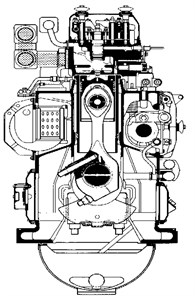Engine type Inline, CI Number of cylinders 6 Charging system Turbo Cylinder bore/stroke [mm] 200/240 Displacement [cm3] 7540 Compression ratio [-] 12.7 Mean piston velocity [m/s] 6 Rated effective power [kW/1 cyl.] for 750 rpm 72 Mean effective pressure [MPa] 1.52 Fuel injection pressure [MPa] 24.5 Combustion pressure [MPa] 11

Fig. 1a) The functional diagram of the measurement test stand and b) the location of the accelerometers and c) microphones in the research object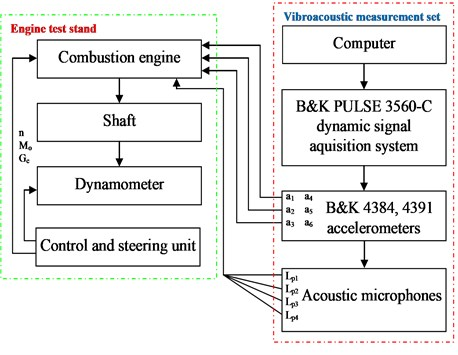a)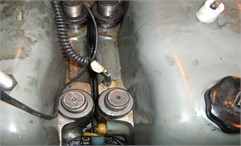b)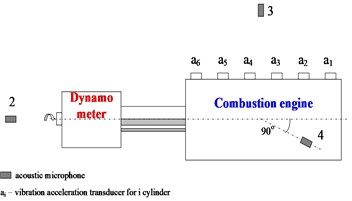c)

The measurement points, their location and engine operating conditions were selected according to the following regulations for marine applications [1, 10, 11]: IMO A.468 (XII) (Directive 2003/10/WE), ISO 2923:2001 International Standard and Polish Standard PN-W-01350-1 to PN-W-01350-7:1996. The vibration acceleration measurement points were located on the engine heads (Fig. 1). The points were selected according to the principle that the sensor should be placed closest to the point where the tested process-related vibration signal is generated. The measurement signals obtained from the accelerometers (Bruel & Kjær 4384 and 4391) and microphones (Bruel & Kjær 4189 A-021) were fed to the amplifiers, in which they were amplified and normalized. They were fed to analog inputs of the data acquisition card. The recording of the vibroacoustic parameters was performed with the use of the Bruel & Kjær PULSE 3560 measurement set [1, 12]. The technical specifications of the measurement set and the vibroacoustic transducers are described in .

The research utilizing vibration and acoustic methods was performed as active experiment, meaning a deliberate modification of the input parameters and observation of their impact on the output parameters [1, 12-15]. The measurements were conducted for different values of the engine speed and torque, according to the following operational characteristics: ${y}_{1}=f\left({N}_{e}\right)$ – propeller screw characteristics; ${y}_{2}=f\left(n,{M}_{o}=const\right)$ – speed characteristics under constant torque; ${y}_{3}=f\left({M}_{o},n=const\right)$ – load characteristics.

The following main diagnostic parameters were taken into consideration in the signal analyses : the parameters of the main processes (fuel injection ${p}_{inj}$ and cylinder ${p}_{c}$ pressure, fuel consumption ${G}_{e}$, ${g}_{e}$ and overall efficiency $\eta$) and the parameters of the vibroacoustic processes (acoustic pressure ${L}_{p}$ and vibration accelerations ${a}_{i}$). The cylinder pressure, fuel injection pressure, acoustic pressure, vibration accelerations and other engine parameters were measured simultaneously on a continuous basis. The constant value of the engine coolant temperature was maintained for all stationary engine operating conditions. Numbers and ranges of engine speeds and torques were selected from the effective area of operating parameters. The following engine speeds [rpm] were selected: 400 (idle run), 450, 500, 550, 600, 650, 700, 750. The effective power values ${N}_{e}/{N}_{e,nominal}$ [%] were: 0.1; 0.25; 0.5; 0.75; 1.0; and the maximum torque for the full power characteristics ($n/{n}_{nominal}=$ 0.53; 0.6; 0.67; 0.73; 0.8; 0.87; 0.93; 1.0 .

The analysis of the operating conditions considered in the research process has confirmed the optimum ranges of the engine operational characteristics in terms of best specific fuel consumption: 223-458 g/kW·h (for propeller screw characteristics), 230-594 g/kW·h (load characteristics) and 314-394 g/kW·h (partial power characteristics). The above values are equal to the conditions of engine operation of the overall efficiency range of 18-37 % .

## 3. Assessment of measurement results

The observation of the values of mean indicated pressure of the research object for each cylinder and for specified engine speeds indicated stable conditions of the engine working cycles in terms of diagnostic accuracy and repeatability of selected processes as well as their estimators for further calculations (Fig. 2).

Fig. 2Differences of mean indicated pressure between engine cylinders for selected engine speed and brake horse power Nh= 270 kW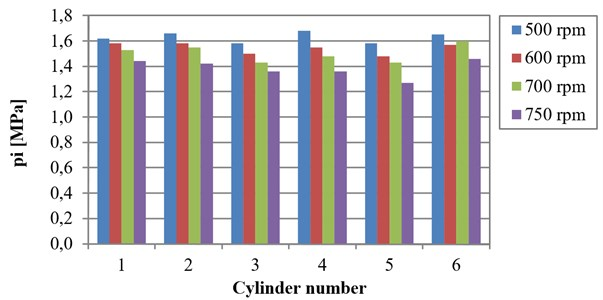When the engine effective power rises the equivalent sound pressure values also increase (Fig. 3). Comparing the differences obtained for the engine speed and those for the engine torque, a greater dynamic change of the above-mentioned sound parameter was observed when the engine speed increased rather than when the torque estimator increased (10 dB for engine speed and 2 dB for torque estimator), which is why the first of the mentioned parameters is more sensitive to acoustic energy changes as a result of the intensity of the main process energy conversion. When an anomaly occurs during normal engine operation (element or assembly malfunctions or negative changes in the course of the process), the observation of the acoustic point estimators changes for constant speed and in some cases might give more stable and accurate results related to the machine technical condition.

Fig. 3Functional relation between the equivalent value of the sound pressure level with the A correction and effective power for the engine operating conditions defined by propeller screw and load a) and partial power b) characteristics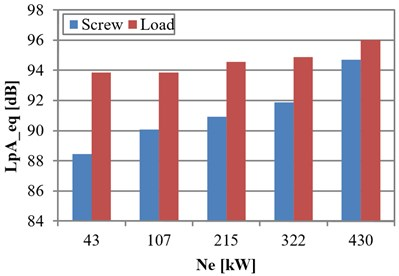a)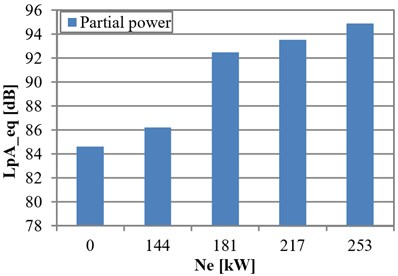b)

In the next step of the analyses, the vibration accelerations were used to determine the environmental impact of a marine diesel engine according to the criteria of proper combustion process and identification of the changes of the optimum values of the overall efficiency and exhaust emissions. Simple vibration point estimators were selected: root mean square (RMS) value ${u}_{sk}$, peak value ${u}_{sz}$ and peak-to-peak value ${u}_{rozst}$, whose definitions are presented as follows [1, 6]:

1
${u}_{sk}={u}_{sk}\left(\theta \right)={\left[\frac{1}{T}{\int }_{0}^{T}{u}^{2}\left(t,\theta \right)dt\right]}^{1/2},$
2
${u}_{sz}={u}_{sz}\left(\theta \right)=E\left\{\underset{0
3
${u}_{rozst}={u}_{rozst}\left(\theta \right)=\left|{u}_{max}-{u}_{min}\right|,$

where: $E\left\{\right\}$ – operator of the mean value after possible peak values of the signal, $t$ – dynamic period, $\theta$ – object life cycle, $T$ – signal period, $u$ – instantaneous signal value.

There is no doubt that the courses of each of the main processes are impactful on the fuel energy transfer (combustion process) and the quality of its conversion into mechanical work. If, for example, two time courses of the injection representation of the injection process in the vibration acceleration signal are recorded at the highest intensity and are transformed in the time and frequency domain with the help of Discrete Wavelet Transform , the change in the energy will take place, when two injector assemblies of different technical condition (a new product and a product with boundary operational ability) are compared (Fig. 4).

The applied wavelet analysis was used deliberately as it ensured simultaneous presentation of the time and frequency related properties of the analyzed signal. As for the Fourier transform, the change of domain from time to frequency results in a loss of information related to the time of the frequency event. To this end the signal is subjected to approximation with elementary functions referred to as wavelets (continuous oscillating courses of even duration and spectrum). The necessary condition, however, is that such functions are mutually orthogonal. This designation of the analysis is decisive of the sought characteristics assigned to the course of the measurement signal. If the signal is non-stationary, such a procedure is justified as the time resolution of the transformation may change (depending on the wavelet frequency). The decomposition of the signal includes the basic course and its details shifted in time of different width in the timeline. Based on the calculated coefficients of compression and shifts in the timeline of all wavelets, a function composed of two variables comprising the time-frequency characteristics of the signal was built. We distinguish continuous wavelet transform (CWT) and discrete wavelet transform (DWT). The CWT is similar to the short-term Fourier transform (the signal is divided into segments using a time window being a wavelet function, subsequently subjected to the FFT evaluation). The selection of the DWT evaluation was substantiated by the difficulties related to the CWT analysis, particularly in terms of analytical solution for more sophisticated signals.

Fig. 4Wavelet transform representation of the vibration acceleration signal for a diesel engine fuel injection for: a new injector a) and that with boundary operational ability (pinj= 80 MPa, tinj= 0.8 ms)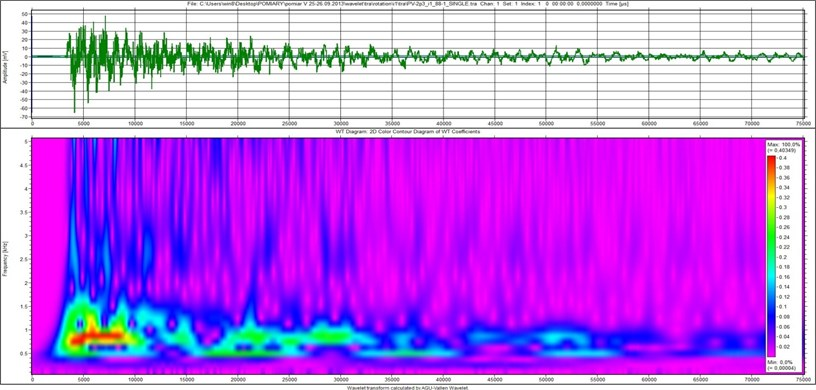a)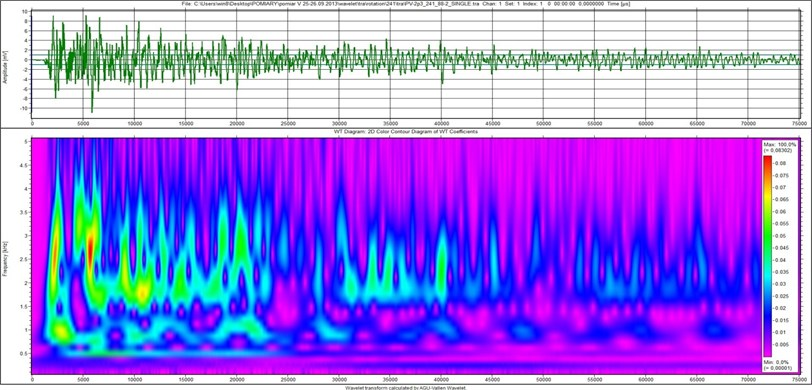b)

The shape of the time window distributed in a given area on the time-frequency platform plays a key role in the case of short-term Fourier transform. The product of the window width in the time and frequency domain is a constant value for a given window. In the wavelet method, the dimensions of the time-frequency window are a function of its position on the time-frequency platform .

The observation of the state of knowledge in signal analyses clearly indicates that it is this particular type of analysis that is the subject of research for scientists seeking more accurate technical object condition diagnostic tools [3-9]. In the scientific works one can observe various applications of the wavelet analysis, starting from engineering applications (roller bearings failure identification in induction motors, inspection of selected parameters of an object, identification of material failures, analysis of wear in kinematic pairs), medical (in cardiology for evaluation of the heart rate variability) or in research on economic processes and their variability, to name only a few. Also, in combustion engines one can observe scientific activity in terms of application of this type of signal analysis for more accurate exploration of the parameter variability. The authors [3-9] applied this tool in the evaluation of the variables of the process of fuel injection in a diesel engine allowing for the structural and operational parameters as well as the injector wear while the source of the diagnostic information was sought with vibroacoustic signal. The example results of analyses presented in Fig. 4 required parameterization of the qualitative relations, which has been described further in the paper.

The same influence is observed when we take into account the injection process conditions (the value of the injection pressure and its quality connected with the injector design). When the first of these characteristics is higher, one can observe quality and quantity changes in the course of the process, which increases the vibration acceleration point estimators, calculated for each time portion of the injection process (Fig. 5).

Fig. 5Relative change of selected vibration acceleration point estimators for diesel oil injection process when the injection pressure increases and for different injectors (pinj= 35 MPa, 90 MPa, tinj= 0.7 ms)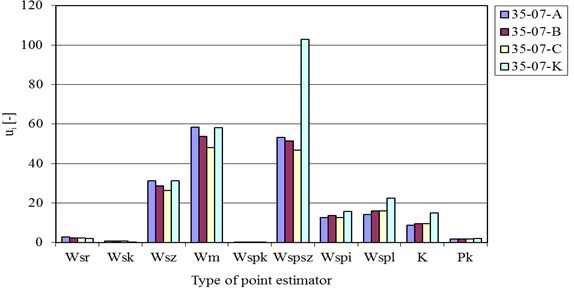a)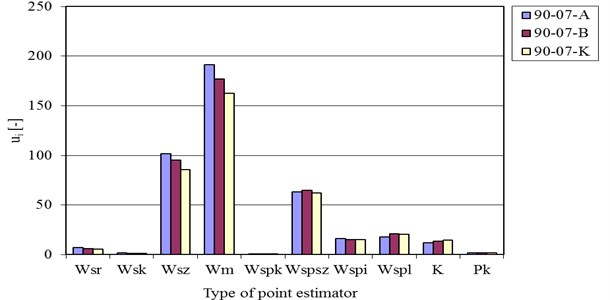b)

The analyses of time course of vibration accelerations for different engine operating conditions (propeller screw, load and partial power characteristics) have confirmed that there are explicit functional relations between the combustion process changes and the one-directional changes of the vibration signal quality and quantity. The increase in the engine effective power resulted in a higher amplitude in each working cycle and similar functional correlations between the said types of estimators for the other cylinders. If there is any abnormality in the combustion process or if misfire occurs, there exists a specified representation in the changes of the vibroacoustic amplitude (Figs. 6-8).

Fig. 6Vibration accelerations time-history courses for cycles with the combustion process and with a misfire for the SULZER 6AL 20/24 engine under the conditions of propeller screw characteristics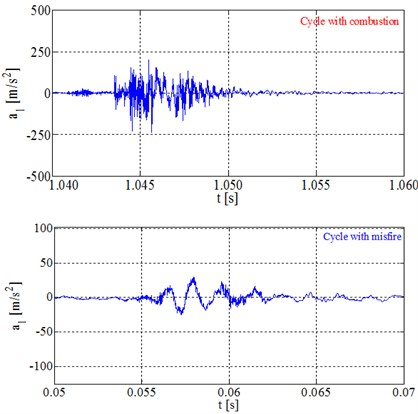a)${N}_{e}/{N}_{e,znam.}=$10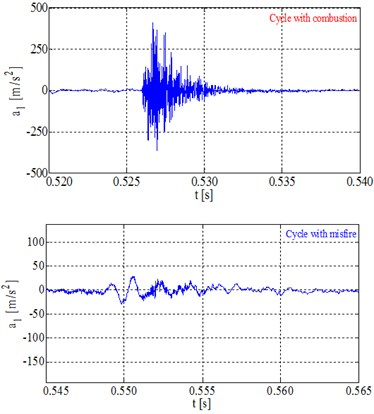b)${N}_{e}/{N}_{e,znam.}=$50

Fig. 7Vibration accelerations point measures for cycles with the combustion process and with a misfire for cylinder 1 of the SULZER 6AL 20/24 engine under the conditions of propeller screw characteristics and at Ne/Ne,znam.= 10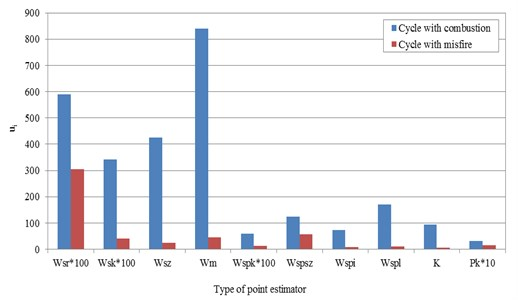## 4. Proposition of combustion process diagnostics algorithm for maritime diesel engine basing on vibration acceleration signal features

The algorithm is in fact a procedure leading to a solution of a problem, determining the individual elementary actions that are to be performed. The underlying characteristics of the algorithm are its correctness, explicitness, finiteness and time and memory efficiency. In the equations of state of a dynamic object we distinguish the following types of courses:

– Input $u\left(t\right)=\left[{u}_{1}\left(t\right),{u}_{2}\left(t\right),\dots ,{u}_{m}\left(t\right)\right]$ as an external impact on the object;

– Output $y\left(t\right)=\left[{y}_{1}\left(t\right),{y}_{2}\left(t\right),\dots ,{y}_{n}\left(t\right)\right]$ as the impact of the object on the environment;

– Coordinates of state $x\left(t\right)=\left[{x}_{1}\left(t\right),{x}_{2}\left(t\right),\dots ,{x}_{p}\left(t\right)\right]$, affecting the type of transformation of input into output;

– Distortions $d\left(t\right)=\left[{d}_{1}\left(t\right),{d}_{2}\left(t\right),\dots ,{d}_{r}\left(t\right)\right]$, as unknown types of inputs;

– Damage $f\left(t\right)=\left[{f}_{1}\left(t\right),{f}_{2}\left(t\right),\dots ,{f}_{s}\left(t\right)\right]$.

Fig. 8Vibration accelerations point measures for cycles with combustion process and with a misfire for cylinder 1 of the SULZER 6AL 20/24 engine under the conditions of propeller screw characteristics and at Ne/Ne,znam.= 50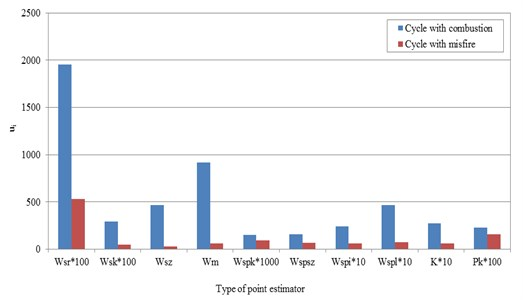The dynamic system is described by the equations of state and outputs:

4
$\stackrel{˙}{x}\left(t\right)=\phi \left[x\left(t\right),u\left(t\right),d\left(t\right),f\left(t\right)\right],$
5
$y\left(t\right)=\psi \left[x\left(t\right),u\left(t\right),d\left(t\right),f\left(t\right)\right].$

The state of the object is defined by a malfunction occurring suddenly or gradually, aging or wear as well as other design or operational changes resulting in the change of object operation. In the case of process diagnostics, the models refer to the evaluation of the functional relations among the symptoms and damage or states of the process. The above malfunctions appear in the form of changes in the values of the object outputs and the physical coefficients of equations of motion. The algorithm must be based on the evaluation of the parameters of individual process changes. Based on the course of the change of the process, the value of the feature of that variable is determined by the following actions: process variable → calculation of a parameter → obtainment of parameter value → evaluation of parameter value → obtainment of the diagnostic signal. In the next stage, a parameterization and comparison of the obtained actual values with the reference estimates (known for the correct state of the object or the course of the process) is performed. Hence, the identification of the malfunction is possible, consisting in determination of the extent of the malfunction and its variation in time and amplitude. For a given malfunction, a subset of diagnostic signals related to this malfunction is determined. The detection-based evaluation of the process abnormalities for analyses based on recognition of signals pertains to the reproduction of the state of the object with reference areas within diagnostic signals (Fig. 9).

The algorithm of the evaluation of the injection process and combustion in the investigated marine diesel engine covers the following procedures:

– intermittent or on-going diagnosis;

– measurement of selected physical quantities sensitive to the tested process changes in the object;

– determination of a set of values of diagnostic parameters;

– comparative evaluation in the domain: diagnostic parameters–reference values separately for the injection and combustion process estimates in individual engine cylinders (referred to the set work area);

– identification of the malfunction and its intensity;

– development of the matrices of states and their location and intensity;

– analysis of the source of malfunction of the analyzed dynamic processes;

– qualification of usability and non-usability of an object in relation to the process functionality (three values);

– conclusion on the technical condition, impact of the malfunction on the structure and its operational characteristics.

Fig. 9The algorithm of failures detection in injection and combustion processes runs at research maritime diesel engine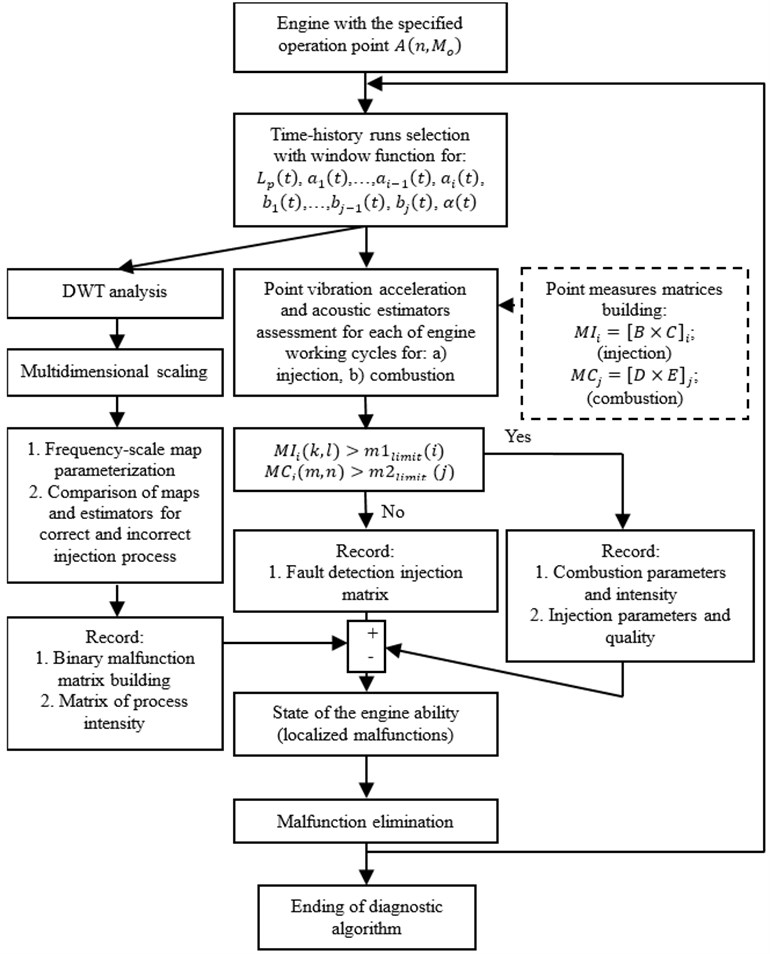## 5. Conclusions

The presented studies concerning the operation of a marine diesel engine used in sea transport applications indicate scientific problems of explicit and reliable evaluation of changes in the machine processes in real time based on more complex non-stationary conditions in operation at sea. The authors of the paper have shown that there is an additional tool for accurate diagnosis of combustion engines used in such vessels that might be applied in diagnostics of the machine technical condition, both for constant values of the engine effective power and under the conditions forcing a change of parameters in time. Empirical research is a method of determining the reliability of vibration and acoustic methods and their sensitivity to engine structural and functional parameter changes with overall efficiency fluctuations, following different energy transformations. Equivalent functional relations between the engine operational parameters and the vibration and acoustic point estimators were defined and obtained from the recorded signals and analytical assumptions. The advantage of such analyses is visible when one allows for the dependence of the environment pollution, intensity of energy propagation, transport safety and optimization of fuel energy consumption on the quality and correctness of dynamic processes taking place in a machine under operation. The presented characteristics and reliable estimators support the application of the method for such a diagnosis and enable determining the extent of impact of marine engines on the global share in air pollution [17-21] and energy conversion efficiency for marine vessels under real operating conditions for which malfunctions may occur in normal working cycles. These analyses also constitute basic data for the ongoing process of malfunction monitoring and the quantitative assessment of these malfunctions and their potential impact on the surrounding systems and organisms.

Wavelet Transform representation of the vibration acceleration signal for a diesel engine fuel injection, for a new injector and that with boundary operational ability, becomes the accurate analytical tool for quality of the injection process run in such type of engines and different object and environmental parameters. It is useful with parameterization of a process, done with the help of process point estimators and their different functions. Vibration acceleration signal point measures and their characteristics were more sensible for negative changes in combustion runs. At the end of a paper, authors were built the algorithm of such faults detection, on the basis of the specified diagnosis procedures application (sound and vibration parameters, analyses in domain of: time, process values and DWT).